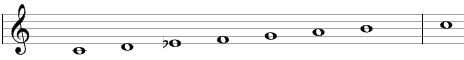# Melodic ascending minor scale

The melodic ascending minor scale is a secondary heptatonic scale that can be built using the following steps between notes: 1, ½, 1, 1, 1, 1, ½.

An example of the melodic ascending minor scale is C, D, Eb, F, G, A, B. This example is shown below in traditional notation and in guitar tablature notation.This scale is essentially the standard major or Ionian scale, but with a lowered third (a minor third, the Eb in the example above).

This scale was commonly used as the minor scale when going up together with the melodic descending minor scale when going down. More recently, the descending minor scale (same as the natural minor scale) is commonly used in both directions.

## Modes of the melodic ascending minor scale

The following are modes of the melodic ascending minor scale.

1. Melodic ascending minor, with steps 1, ½, 1, 1, 1, 1, ½ (C, D, Eb, F, G, A, B in the example above).
2. Phrygian with a sharp sixth, with steps ½, 1, 1, 1, 1, ½, 1 (D, Eb, F, G, A, B, C).
3. Lydian with a sharp fifth, with steps 1, 1, 1, 1, ½, 1, ½ (Eb, F, G, A, B, C, D).
4. Lydian-Mixolydian, with steps 1, 1, 1, ½, 1, ½, 1 (F, G, A, B, C, D, Eb).
5. Major-minor, with steps 1, 1, ½, 1, ½, 1, 1 (G, A, B, C, D, Eb, F).
6. Locrian with a sharp second, with steps 1, ½, 1, ½, 1, 1, 1 (A, B, C, D, Eb, F, G).
7. Altered (Locrian with a flat fourth), with steps ½, 1, ½, 1, 1, 1, 1 (B, C, D, Eb, F, G, A).

## Three-note chords on the melodic ascending minor scale

The following are common triads built on the notes of the melodic ascending minor scale.

• On the root of the scale (on the tonic): minor chord (e.g., Cm composed of C, Eb, G) or suspended chords (Csus4 = C, F, G and Csus2 = C, D, G).
• On the second note (on the supertonic): minor chord (Dm = D, F, A) or suspended chord (Dsus4 = D, G, A).
• On the third note (on the mediant): augmented chord (Ebaug = Eb, G, B).
• On the fourth note (on the subdominant): major chord (F = F, A, C) or suspended chord (Fsus2 = F, G, C).
• On the fifth note (on the dominant): major chord (G = G, B, D), augmented chord (G, B, Eb) or suspended chords (Gsus4 = G, C, D and Gsus2 = G, A, D).
• On the sixth note (on the submediant): diminished chord (Adim = A, C, Eb).
• On the seventh note (on the leading tone): augmented chord (Baug = B, Eb, G) or diminished chord (Bdim = B, D, F).

## Four-note chords on the melodic ascending minor scale

The following are seventh chords built on the notes of the melodic ascending minor scale.

• On the first note: minor-major seventh chord (e.g., Cmmaj7 composed of C, Eb, G, B).
• On the second note: minor seventh chord (Dmin7 = D, F, A, C).
• On the third note: augmented major seventh chord (Ebmaj7#5 = Eb, G, B, D).
• On the fourth note: dominant seventh chord (F7 = F, A, C, Eb).
• On the fifth note: dominant seventh chord (G7 = G, B, D, F) or augmented seventh chord (G7#5 = G, B, Eb, F).
• On the sixth note: half-diminished seventh chord (Am7b5 = A, C, Eb, G).
• On the seventh note: half-diminished seventh chord (Bm7b5 = B, D, F, A) or augmented seventh chord (B7#5 = B, Eb, G, A).

## Intervals on the melodic ascending minor scale

The melodic ascending minor scale is composed of the following intervals.

• A major second, e.g., the interval between C and D is equal to two semitones.
• A minor third, the interval between C and Eb is equal to three semitones.
• A perfect fourth, the interval between C and F is equal to five semitones.
• A perfect fifth, the interval between C and G is equal to seven semitones.
• A major sixth, the interval between C and A is equal to nine semitones.
• A major seventh, the interval between C and B is equal to eleven semitones.

Scale, Scale (index)

### Filtered HTML

• Freelinking helps you easily create HTML links. Links take the form of [[indicator:target|Title]]. By default (no indicator): Click to view a local node.